## Applications of Percents

Percents can be used in many types of problems and situations. The following applications are the most common basic types.

To determine percent of a number, change the percent to a fraction or decimal (whichever is easier for you) and multiply. Remember: The word of means multiply.

Find the percents of the following numbers.

What is 20% of 80?

What is 15% of 50?

What isof 18?

What is 70% of 20?

Using fractions,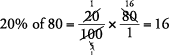Using decimals,

20% of 80 = 0.20 × 80 = 16.00 = 16

Using fractions,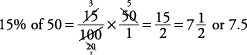Using decimals,

15% of 50 = 0.15 × 50 = 7.5

Using fractions,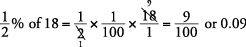Using decimals,Using fractions,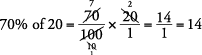Using decimals,

70% of 20 = 0.70 × 20 = 14

One method to find what percent one number is of another is the division method. To use this method, simply take the number after the of and divide it into the number next to the is. Then change the answer to a percent.

Find the percentages.

20 is what percent of 50?

27 is what percent of 90?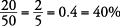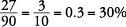Another method to find what percent one number is of another is the equation method. Simply turn the question word‐for‐word into an equation. For what, substitute the letter x; for is, substitute an equal sign (=); for of, substitute a multiplication sign (×). Change percents to decimals or fractions, whichever you find easier. Then solve the equation.

Change each of the following into an equation and solve.

10 is what percent of 50?

15 is what percent of 60?

10 is what percent of 50?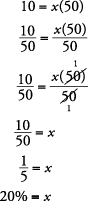Therefore, 10 is 20% of 50.

15 is what percent of 60?Therefore, 15 is 25% of 60.

You can also use the division method to find a number when a percent of it is known. To use this method, simply take the number of percent, change it into a decimal or fraction, and divide that into the other number.

You could also use the equation method as discussed previously. Simply turn the question word‐for‐word into an equation. For what, substitute the letter x; for is, substitute an equal sign (=); for of, substitute amultiplication sign (×). Change percents to decimals or fractions, whichever you find easier. Then solve the equation.

Find the number.

15 is 50% of what number?

20 is 40% of what number?Find the number.

30 is 20% of what number?

40 is 80% of what number?

30 is 20% of what number?So 30 is 20% of 150.

40 is 80% of what number?So 40 is 80% of 50.

Another simple method commonly used to solve any of the three types of percent problems is the proportion method (also called the is/of method).

First set up a blank proportion and then fill in the empty spaces by using the following steps.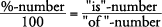Whatever is next to the percent (%) is put over 100. (The word what is the unknown, or x.)

Whatever comes immediately after the word of goes on the bottom of one side of the proportion.

Whatever is left (comes next to the word is) goes on top, on one side of the proportion.

Then solve the problem.

30 is what percent of 50?

Set up a blank proportion.30 is what percent of 50?

Step 1:Step 2: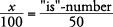Step 3: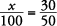Step 4:In this particular problem, however, it can be observed quickly that, so solving mechanically as shown is not time effective.

The proportion method works for the three basic types of percent questions:

40 is what percent of 200?

(which is the same as what percent of 200 is 40?)

60 is 20% of what number?

(which is the same as 20% of what number is 60?)

What number is 15% of 30? (In this type, simply multiplying the numbers is probably easier.)

(which is the same as 15% of 30 is what number?)

Solve using the proportion method.

40 is what percent of 200?

60 is 20% of what number?

What number is 15% of 30?

40 is what percent of 200?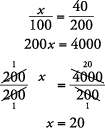So 40 is 20% of 200.

60 is 20% of what number?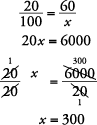So 60 is 20% of 300.

What number is 15% of 30?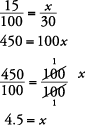So 4.5 is 15% of 30.

To find percent change (increase or decrease), use this formula:Find the percent change.

a

What is the percent decrease of a \$500 item on sale for \$400?

change = 500 – 400 = 100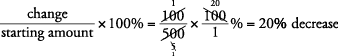What is the percent increase of Jon's salary if it went from \$150 a month to \$200 a month?

change = 200 – 150 = 50What is the percent change from 2,100 to 1,890?

change = 2,100 – 1,890 = 210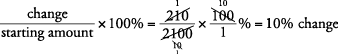Note: The terms percentage rise, percentage difference, and percentage change are the same as percent change.